Publicité

# Algorithm Class at KPHB C, c++, ds,cpp,java,data structures training institute in kphb, kukatpally, hyderabad, course

12 May 2015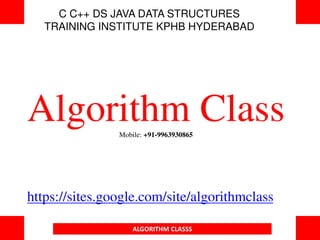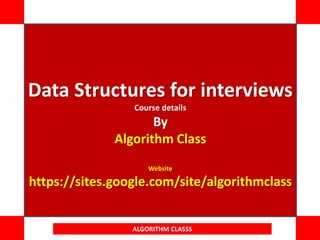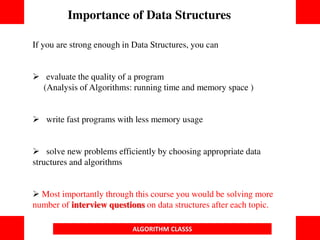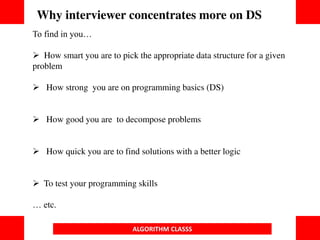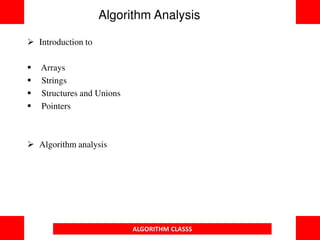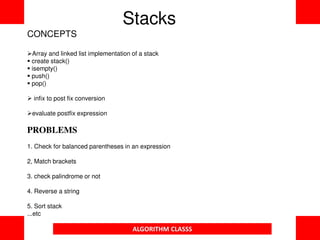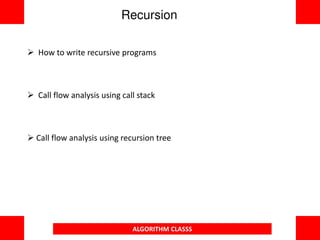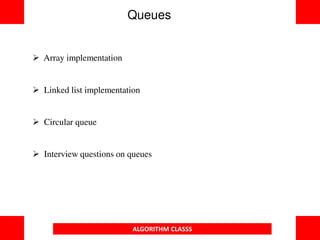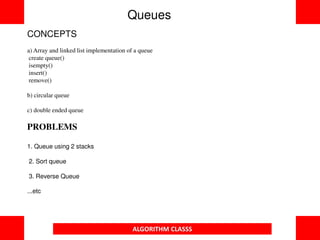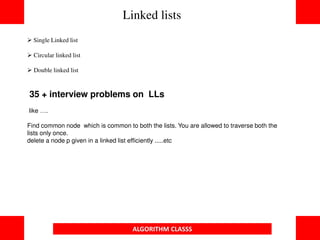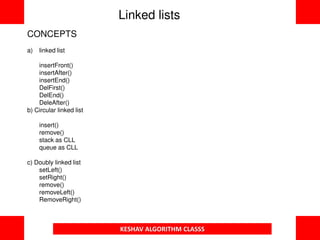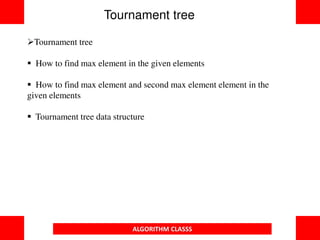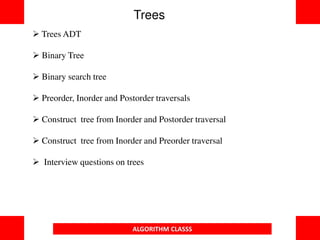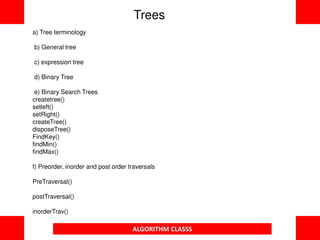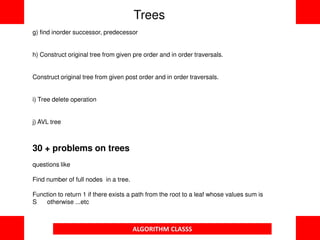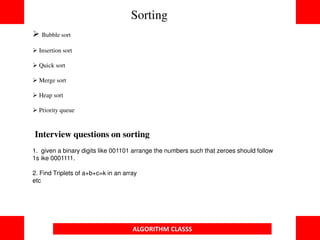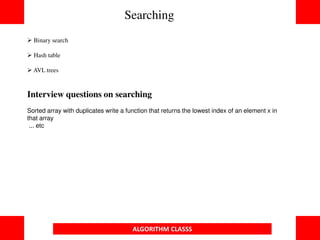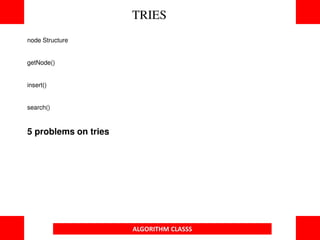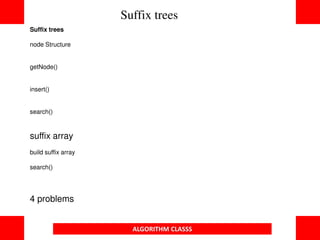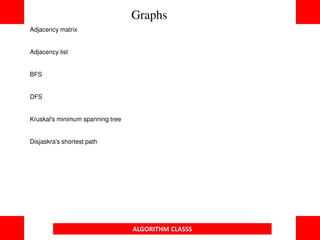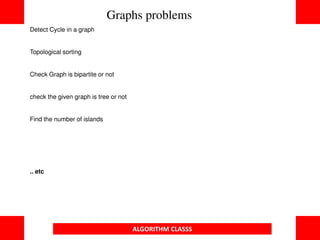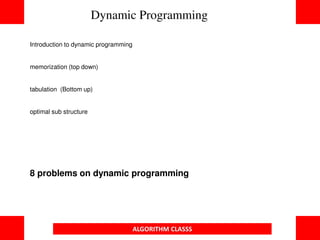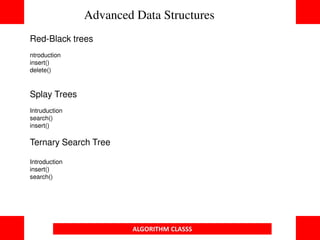1 sur 24
Publicité

### Algorithm Class at KPHB C, c++, ds,cpp,java,data structures training institute in kphb, kukatpally, hyderabad, course

1. C C++ DS JAVA DATA STRUCTURES TRAINING INSTITUTE KPHB HYDERABAD Algorithm ClassMobile: +91-9963930865 https://sites.google.com/site/algorithmclass ALGORITHM CLASSS
2. ALGORITHM CLASSS Data Structures for interviews Course details By Algorithm Class Website https://sites.google.com/site/algorithmclass
3. If you are strong enough in Data Structures, you can  evaluate the quality of a program (Analysis of Algorithms: running time and memory space )  write fast programs with less memory usage  solve new problems efficiently by choosing appropriate data structures and algorithms  Most importantly through this course you would be solving more number of interview questions on data structures after each topic. Importance of Data Structures ALGORITHM CLASSS
4. To find in you…  How smart you are to pick the appropriate data structure for a given problem  How strong you are on programming basics (DS)  How good you are to decompose problems  How quick you are to find solutions with a better logic  To test your programming skills … etc. Why interviewer concentrates more on DS ALGORITHM CLASSS
5.  Introduction to  Arrays  Strings  Structures and Unions  Pointers  Algorithm analysis Algorithm Analysis ALGORITHM CLASSS
6. CONCEPTS Array and linked list implementation of a stack  create stack()  isempty()  push()  pop()  infix to post fix conversion evaluate postfix expression PROBLEMS 1. Check for balanced parentheses in an expression 2, Match brackets 3. check palindrome or not 4. Reverse a string 5. Sort stack ...etc Stacks ALGORITHM CLASSS
7.  How to write recursive programs  Call flow analysis using call stack  Call flow analysis using recursion tree Recursion ALGORITHM CLASSS
8. Queues  Array implementation  Linked list implementation  Circular queue  Interview questions on queues ALGORITHM CLASSS
9. CONCEPTS a) Array and linked list implementation of a queue create queue() isempty() insert() remove() b) circular queue c) double ended queue PROBLEMS 1. Queue using 2 stacks 2. Sort queue 3. Reverse Queue ...etc Queues ALGORITHM CLASSS
10.  Single Linked list  Circular linked list  Double linked list 35 + interview problems on LLs like …. Find common node which is common to both the lists. You are allowed to traverse both the lists only once. delete a node p given in a linked list efficiently .....etc Linked lists ALGORITHM CLASSS
11. CONCEPTS a) linked list insertFront() insertAfter() insertEnd() DelFirst() DelEnd() DeleAfter() b) Circular linked list insert() remove() stack as CLL queue as CLL c) Doubly linked list setLeft() setRight() remove() removeLeft() RemoveRight() Linked lists KESHAV ALGORITHM CLASSS
12. Tournament tree  How to find max element in the given elements  How to find max element and second max element element in the given elements  Tournament tree data structure Tournament tree ALGORITHM CLASSS
13.  Trees ADT  Binary Tree  Binary search tree  Preorder, Inorder and Postorder traversals  Construct tree from Inorder and Postorder traversal  Construct tree from Inorder and Preorder traversal  Interview questions on trees Trees ALGORITHM CLASSS
14. a) Tree terminology b) General tree c) expression tree d) Binary Tree e) Binary Search Trees createtree() setleft() setRight() createTree() disposeTree() FindKey() findMin() findMax() f) Preorder, inorder and post order traversals PreTraversal() postTraversal() inorderTrav() Trees ALGORITHM CLASSS
15. g) find inorder successor, predecessor h) Construct original tree from given pre order and in order traversals. Construct original tree from given post order and in order traversals. i) Tree delete operation j) AVL tree 30 + problems on trees questions like Find number of full nodes in a tree. Function to return 1 if there exists a path from the root to a leaf whose values sum is S otherwise ...etc Trees ALGORITHM CLASSS
16.  Bubble sort  Insertion sort  Quick sort  Merge sort  Heap sort  Priority queue Interview questions on sorting 1. given a binary digits like 001101 arrange the numbers such that zeroes should follow 1s ike 0001111. 2. Find Triplets of a+b+c=k in an array etc Sorting ALGORITHM CLASSS
17.  Binary search  Hash table  AVL trees Interview questions on searching Sorted array with duplicates write a function that returns the lowest index of an element x in that array ... etc Searching ALGORITHM CLASSS
18. node Structure getNode() insert() search() 5 problems on tries TRIES ALGORITHM CLASSS
19. Suffix trees node Structure getNode() insert() search() suffix array build suffix array search() 4 problems Suffix trees ALGORITHM CLASSS
20. Adjacency matrix Adjacency list BFS DFS Kruskal's minimum spanning tree Disjaskra's shortest path Graphs ALGORITHM CLASSS
21. Detect Cycle in a graph Topological sorting Check Graph is bipartite or not check the given graph is tree or not Find the number of islands .. etc Graphs problems ALGORITHM CLASSS
22. Introduction to dynamic programming memorization (top down) tabulation (Bottom up) optimal sub structure 8 problems on dynamic programming Dynamic Programming ALGORITHM CLASSS
23. Red-Black trees ntroduction insert() delete() Splay Trees Intruduction search() insert() Ternary Search Tree Introduction insert() search() Advanced Data Structures ALGORITHM CLASSS
24. CONTACT Algorithm Class Nizampet, Hyderabad. Mobile: +91-9963930865 Visit for more details about other courses: https://sites.google.com/site/algorithmclass ALGORITHM CLASSS
Publicité